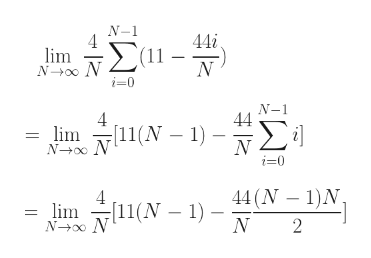# Calculate the limit for the function f(x) = 33 - 11x and interval [2, 6]. Verify your answer by using geometry(Give your answer as a whole or exact number.)

Question
90 views

check_circle

Step 1

We have to essentially evaluate the limit shown on the white board.

[a, b] = [2, 6] Hence, a = 2, b = 6

Let's split into N sub-intervals. Each sub-interval is therefore of length =Δx = (b - a) / N = (6 - 2) / N = 4/N

Step 2

f(x) = 33 - 11x

f(a + iΔx) = f(2 + 4i / N) = 33 - 11(2 + 4i / N) = 11 - 44i/Nhelp_outlineImage TranscriptioncloseN-1 44i N-o0 N i0 N-1 4 = lim No N [11(N - 1) 44 (N 1)N lim11(N) N 2 fullscreen
Step 3

The steps are continued over here. ...

### Want to see the full answer?

See Solution

#### Want to see this answer and more?

Solutions are written by subject experts who are available 24/7. Questions are typically answered within 1 hour.*

See Solution
*Response times may vary by subject and question.
Tagged in

### Limits